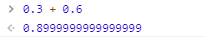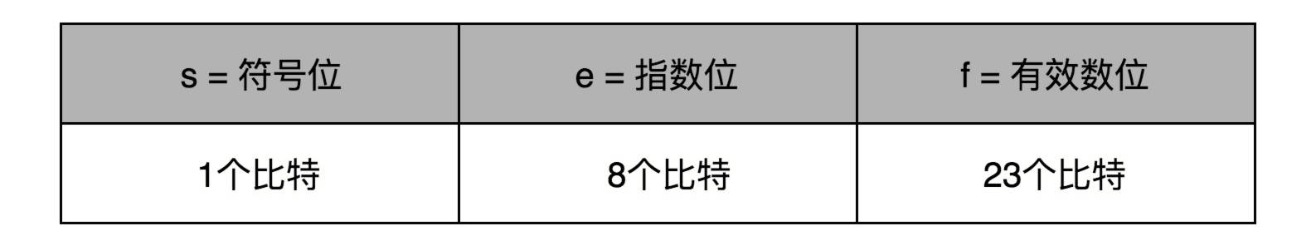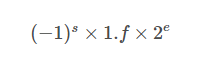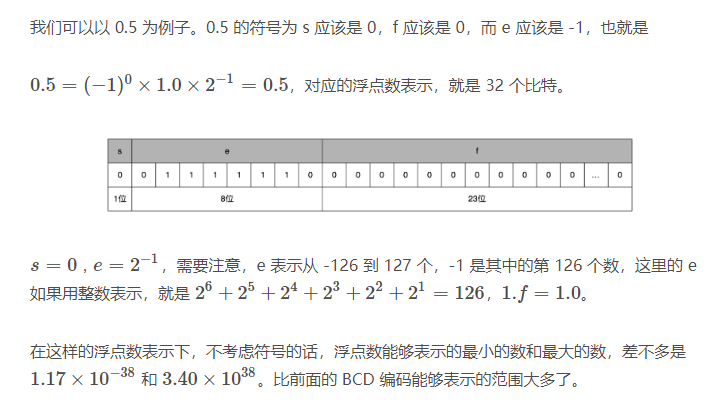# 组成原理|为什么计算机中0.3 + 0.6 等于 0.899999999...？

## 浮点数的不精确性## 浮点数的表示

IEEE的标准，它定义了两个基本的格式：float单精度 和 double双精度

1.符号为表示正负0和1。
2.指数位，因为我们也需要表示很小的数，指数位置需要负数，所以我们在这里用 1～254 映射到 -126～127 这 254个有正有负的数
3.有效数位，是一个 23 个比特组成的有效数位。我们用f来表示。## 浮点数相加的精度误差

float a = 20000000.0f;
float b = 1.0f;
float sum = a + b;
print sum //发现sum的值还是2000万，而不是 200000001




float sum = 0.0f;
for (int i = 0; i < 20000000; i++) {
float x = 1.0f;
sum += x;
}
print sum // sum 约等于1.6777216E7




float sum = 0.0f;
float c = 0.0f;
for (int i = 0; i < 20000000; i++) {
float x = 1.0f;
float y = x - c;
float t = sum + y;
c = (t-sum)-y;
sum = t;
}
print sum;//2000万


posted @ 2019-06-19 09:31  fishers  阅读(2903)  评论(4编辑  收藏  举报